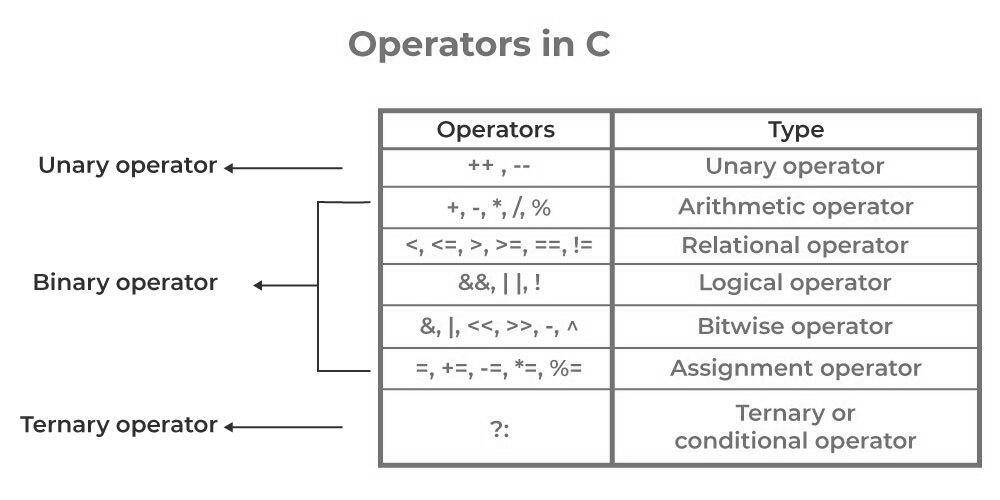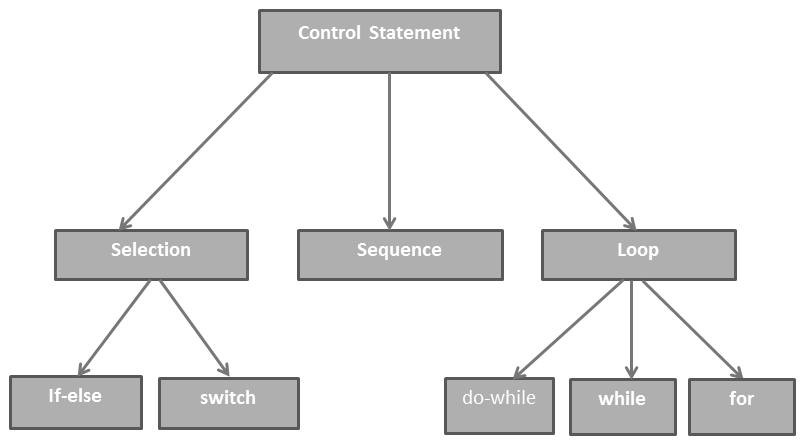# Structured Programming in C

By Notes Vandar

## Introduction to Structured Programming in C

Structured programming in C is a programming paradigm that emphasizes the use of well-defined control structures, such as loops and conditional statements, to organize and simplify code. It is based on the idea that a program should be divided into smaller, modular units, or “structures,” that can be easily understood and maintained.

In C, structured programming is achieved through the use of functions, which are self-contained blocks of code that can be called and reused throughout a program. Functions allow you to divide a program into smaller, more manageable units, and to define clear interfaces between them. This can make it easier to understand and debug a program, as well as to maintain and modify it over time.

To use structured programming in C, you will need to be familiar with basic programming concepts, such as variables, data types, loops, and conditional statements. You will also need to understand how to define and use functions in C, including how to pass arguments to and return values from functions.

Here is an example of a simple function in C that calculates the area of a rectangle:

```float calculate_area(float width, float height) {
return width * height;
}
```

In this example, the function “calculate_area” takes two arguments, “width” and “height,” and returns the product of these two values as the result. The function can be called from anywhere in the program by providing the required arguments and storing the result in a variable.

By using functions and other control structures, such as loops and conditional statements, you can organize your code in a logical and easy-to-understand way, and improve its readability, clarity, and maintainability. Structured programming in C is an important programming skill, and a foundational skill for many other programming tasks, such as object-oriented programming and data structures.

## C Programming and its Features.

C Programming is a popular high-level language developed by Denish Ritchie. It is a structural programming language (i.e supports several control structures) developed for a specific purpose to design the UNIX operating system.

### Features of C language.

1. It is a structural and modular programming language.
2. It is a case-sensitive language.
3. It supports graphics and several mathematical functions.
4. It has several libraries and other functions.

Data type: C supports both numeric as well as alphanumeric data. Frequently used Data types in C are:

[Note: There are more data types which we will discuss later in need]

Variable: Those entities which hold either numeric or alphanumeric values in the program and may change their value throughout the time of program execution are known as variables.

### The rule for writing variables:

1. Variable names should not start will a number. Eg, 1age is invalid, age1 is valid
2. Variable names should not have blank spaces. Eg, if the first name is invalid, the first name is valid
3. Keywords cannot be used as a variable names. Eg, printf = 2; is invalid, num = 2; is valid
4. Uppercase variable names are different from lowercase variable names. Eg, age = 2 is different from AGE = 2

## What are Operators?

Operators: The special symbol or sign used to perform some specific function or operation are called operators.Operators in C Programming

### Types of operators:

#### a) Arithmetic operator

+ , – , * , / , %

Note that Here,  if c = 5/2

The result will be 2 if we initialize ‘c’ as int c.

The result will be 2.5 if we initialize ‘c’ as float c.

If c = 5%2 then the result will be 1. Since % gives the remainder after division.

#### b) Relational operator

> , < , >= , <= , != (not equal to) , == (equal to)

[Note: Here in C language if you want to compare the equal to is referred by == (two equals)]

#### c) Assignment operator

= is an assignment operator.

[Note If a = 2 then it means 2 is assigned to variable a, it does not mean an also has value 2 and both are equal.]

#### d) Logical operator

For logical AND use &&

For logical OR use ||

For logical NOT use!

It is a file in the C library with .h extension and contains several functions declarations and definitions. Such as we use.

#include for standard input output function eg, printf, scanf, etc

#include for mathematical function eg, pow, sin, cos, etc

#include for graphical element eg, circle, rectangle, line, etc

#include for string handling function eg, strcpy(), strlen(),strupr(), etc

#### Sample structure of C program:

```#include
#include
void main ( )
{
getch ( );
}```

Explanation: C program always executes from top left to right bottom, so program execution starts from the header file which is followed by the main( ) function. Remember that main( ) is always executed first. Since main( ) is a function it will return some values so we write void in front of main( ) as a return type which means a null value is returned. getch( ) will hold our output on the screen unless the user presses some keys. It means getting the character. All other statements written inside the main( ) function will be executed sequentially. If you are using void and getch don’t forget to also include conio.h as a header file.

#### The same program can be written as

```#include
int main( )
{
return 0;
}```

Here, since we have written int main( ) The main( ) function should return an integer value so we write return 0 instead of getch( )here. While using int main( ) and return 0, we don’t need to include conio.h

### Output statement:

Output in C program can be displayed by using the ‘printf’ statement.

A) In order to display only characters we can use the following syntax

printf(“Sample text”);

This will display anything that is written inside double quotation marks (“ “)

B) In order to display the values of the variable we can use the following syntax

printf(“format specifier”, list of variables,…);

Example :

```int a = 4, b = 6, c;
c = a+b;
printf(“Sum is %d”, c);```

It will display: Sum is c

[Note that any number of format specifiers and variables can be displayed]

The same program can be displayed as

`printf(“The sum of %d and %d is %d”, a, b, c);`

It will display: Sum of 4 and 6 as 10

[Note: if a, b and c were initialized as a float then every %d should be replaced by %f]

### Input statement:

Input in the C program can be taken by using the ‘scanf’ statement.

In order to take input from the user  we can use the following syntax

scanf(“format specifiers”, &variablename1, &variablename2, ….);

Suppose we want to take a and b as input from the user. To take number input we can write

```int a, b;
scanf(“%d %d”, &a, &b);```

In this example, two numeric variables are initialized as integers. So, we write two %d inside double quotation followed by &variablename. & denotes the address of that variable.

Another example,

If we want to take principal, time, and rate then it can be written as

```float p, t, r;
scanf(“%f %f %f”, &p, &t, &r);```

In this example, three numeric variables are initialized as a float. Since, their values may be in decimal So, we write three %f inside a double quotation followed by &variablename. & denotes the address of that variable.

If we want to take a character or string as input then the first string variable should be initialized as follows:

`char fname;`

Remember if we initialized the variable as ‘char’ then the variable used becomes a string. Since string in C is an array of characters we should suffix variable name with size [size] i.e maximum length of character that the variable can hold.

[Note: While taking string as an input we don’t need to write & in variable name]

Example

```char fname, lname;
scanf(“%s %s”, fname, lname);```

Since variables are initialized as an array of characters we don’t need to mention & while using scanf.

### Control structure in C:

Since C is a structural programming language, we can change the flow of program execution according to the requirement of the user. Following is the control structure used in C.Control structure in C

Important Questions